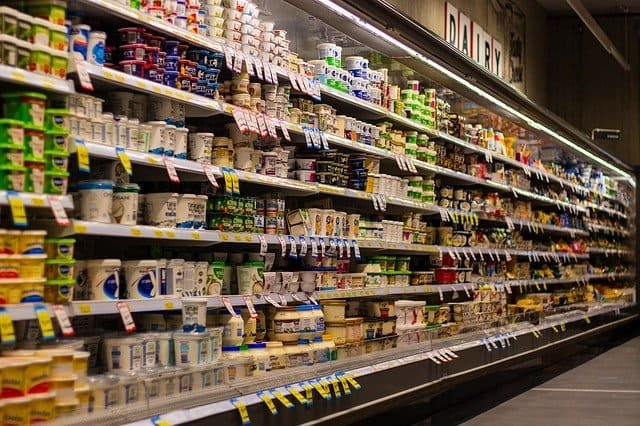Contact & Support : +91 95990 97843   |• Rs. 19
Rs. 20
[Rs.5% OFF]
• Rs. 19
Rs. 20
[Rs.5% OFF]
• Rs. 21
Rs. 22
[Rs.5% OFF]
• Rs. 21
Rs. 22
[Rs.5% OFF]
• Rs. 25
Rs. 25
[Rs.0% OFF]
• Rs. 25
Rs. 25
[Rs.0% OFF]
• Rs. 26
Rs. 27
[Rs.4% OFF]
• Rs. 26
Rs. 28
[Rs.7% OFF]
• Rs. 27
Rs. 30
[Rs.10% OFF]
• Rs. 28
Rs. 30
[Rs.7% OFF]
• Rs. 29
Rs. 30
[Rs.3% OFF]
• Rs. 28
Rs. 30
[Rs.7% OFF]
• Rs. 42
Rs. 45
[Rs.7% OFF]
• Rs. 48
Rs. 50
[Rs.4% OFF]
• Rs. 54
Rs. 55
[Rs.2% OFF]
• Rs. 53
Rs. 55
[Rs.4% OFF]
• Rs. 62
Rs. 65
[Rs.5% OFF]
• Rs. 55
Rs. 65
[Rs.15% OFF]
• Rs. 68
Rs. 70
[Rs.3% OFF]
• Rs. 65
Rs. 75
[Rs.13% OFF]
• Rs. 75
Rs. 78
[Rs.4% OFF]
• Rs. 60
Rs. 80
[Rs.25% OFF]
• Rs. 99
Rs. 105
[Rs.6% OFF]
• Rs. 105
Rs. 108
[Rs.3% OFF]
• Rs. 118
Rs. 140
[Rs.16% OFF]
• Rs. 122
Rs. 146
[Rs.16% OFF]
• Rs. 270
Rs. 281
[Rs.4% OFF]
• Rs. 249
Rs. 320
[Rs.22% OFF]
• Rs. 1350
Rs. 1995
[Rs.32% OFF]